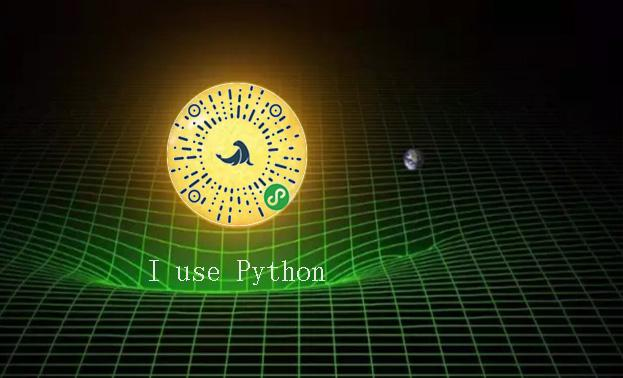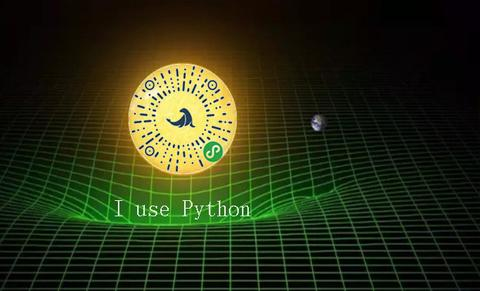1.项目背景：

2.分析步骤：

（1）数据清洗（Data Cleaning）

（2） 探索性可视化（Exploratory Visualization）

（3） 特征工程（Feature Engineering）

（4）基本建模&评估（Basic Modeling& Evaluation）

3.源码（公众号：海豹战队）：

``````# coding: utf-8
# 亲，转载即同意帮推公众号：海豹战队，嘿嘿......
# 数据源可关注公众号：海报战队，后留言：数据

import pandas as pd
filename = '../data/bankloan.xls'
data = pd.read_excel(filename)
data.head()
x = data.drop('违约',axis=1) # 舍弃违约列进行训练
y = data['违约'] # y标签为‘违约’列
# y.unique()
from sklearn.linear_model import LogisticRegression as LR
from sklearn.linear_model import RandomizedLogisticRegression as RLR
rlr = RLR() #建立随机逻辑回归模型，筛选变量
rlr.fit(x, y) #训练模型
rlr.get_support() #获取特征筛选结果，也可以通过.scores_方法获取各个特征的分数
print('通过随机逻辑回归模型筛选特征结束。')
print('有效特征为：%s' % ','.join(x.columns[rlr.get_support()]))
x= x[x.columns[rlr.get_support()]]  # 选择有效特征列进行模型训练
#x.head()
lr = LR() #建立逻辑回归模型
lr.fit(x, y) #用筛选后的特征数据来训练模型
print('逻辑回归模型训练结束。')
print('模型的正确率为：%s' % lr.score(x, y)) #给出模型的平均正确率，本例为81.4%
lr.predict([[10,6,17.3,1.362202]])  # 挑第二个人给预测一下
lr.predict([[10,6,17.3,10.362202]])# 提高第二个人的负债再预测，自己看看差别

# 建议以上代码在jupyter notebook 分段执行，查看结果。``````

yeayee：Python数据分析及可视化实例目录zhuanlan.zhihu.com